# Wolf Dynamics - Multiphysics simulations, optimization, and data analytics

## OPENFOAM VALIDATION CASES

Supersonic flow past a wedge

In this case, we simulate a supersonic flow past a wedge. The inlet Mach number is 3, and the wedge angle is 20 degrees. The exact solution to this problem can be computed using oblique shock relations (or the θ-β-M diagram).

in this validation case, we also compare the dependence of the solution on the mesh resolution.  We also show two methods to capture the shock wave, namely, numerical Schlieren (gradient of density), and numerical shadowgraph (Laplacian of the gradient).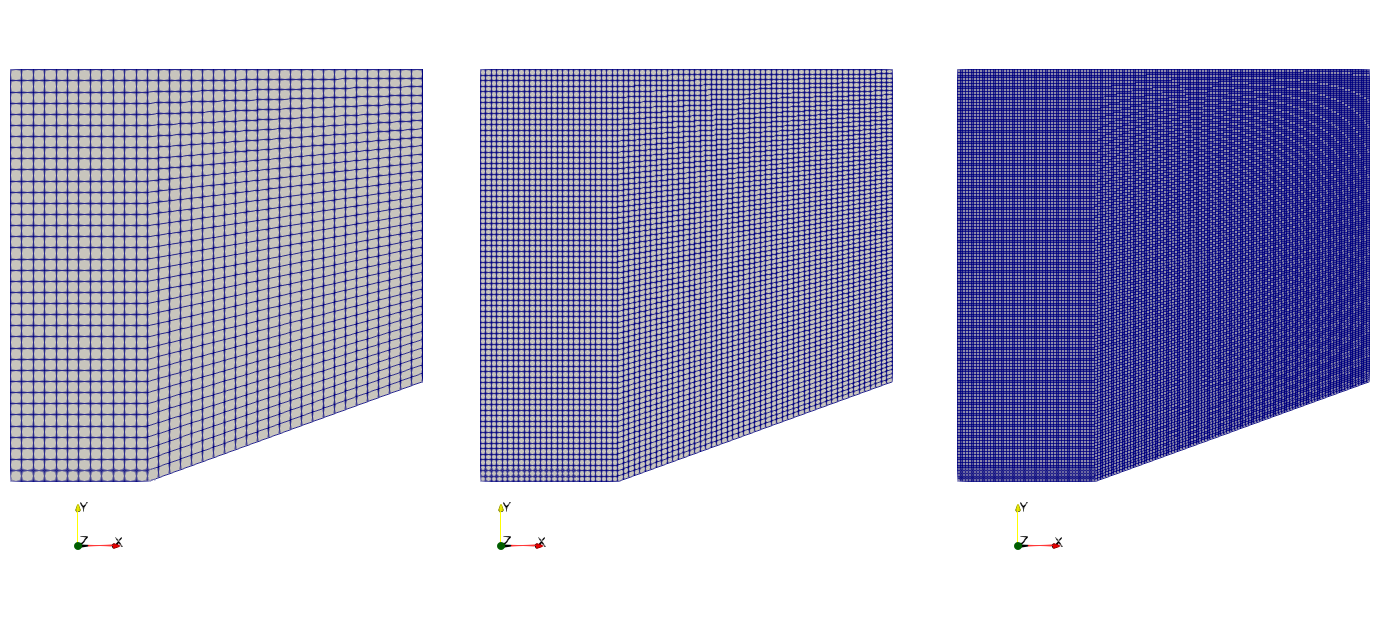Meshes used in this validation study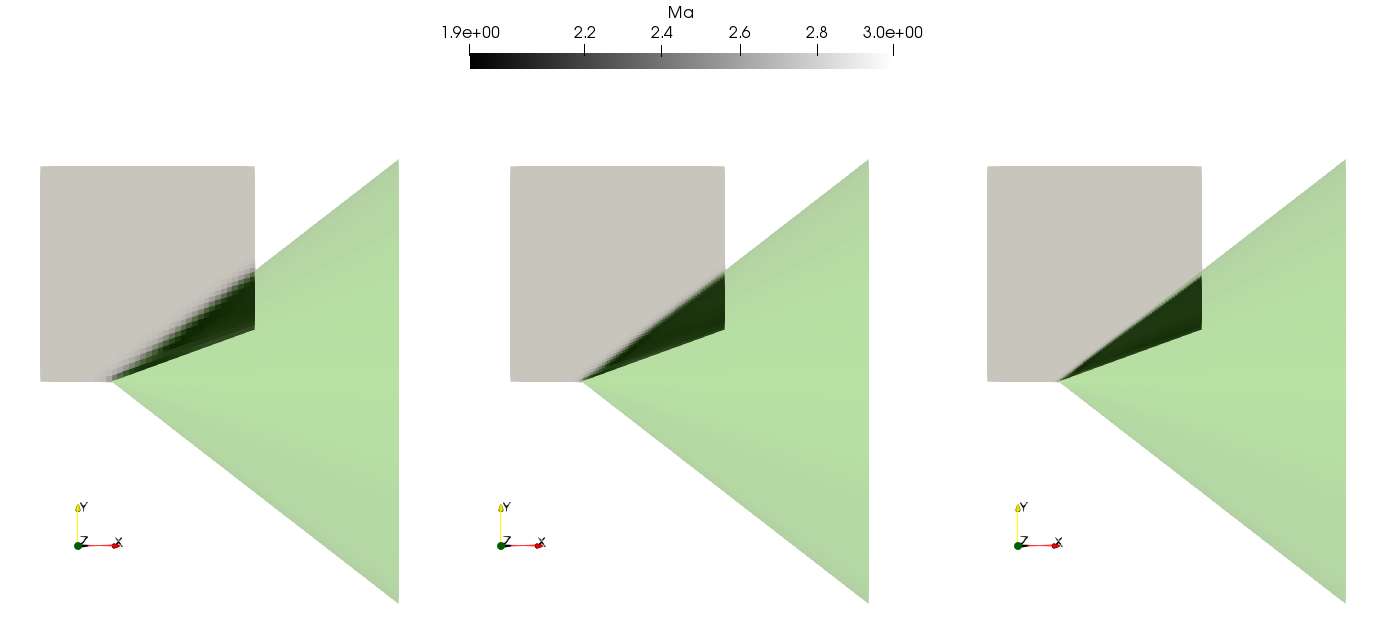Mach number contours and cone showing the exact shock wave turning angle (transparent green surface). From left to right, coarse to finer mesh.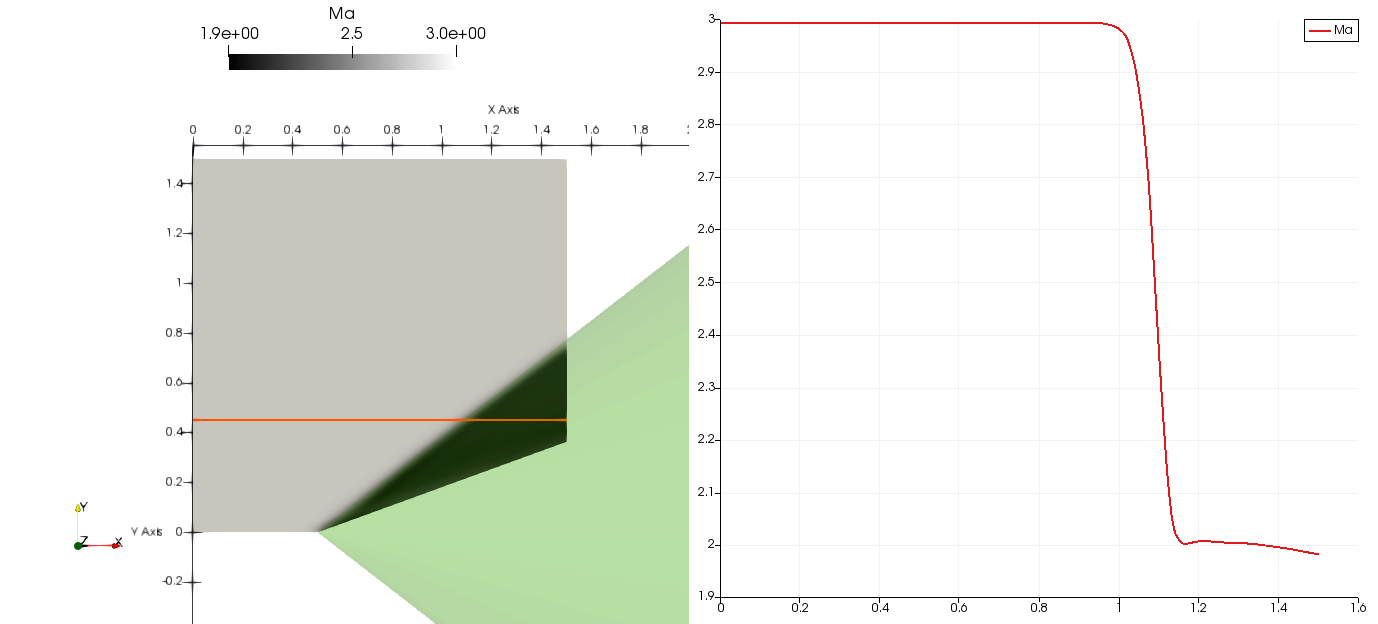Shock wave visualization and Mach number along a line (orange line).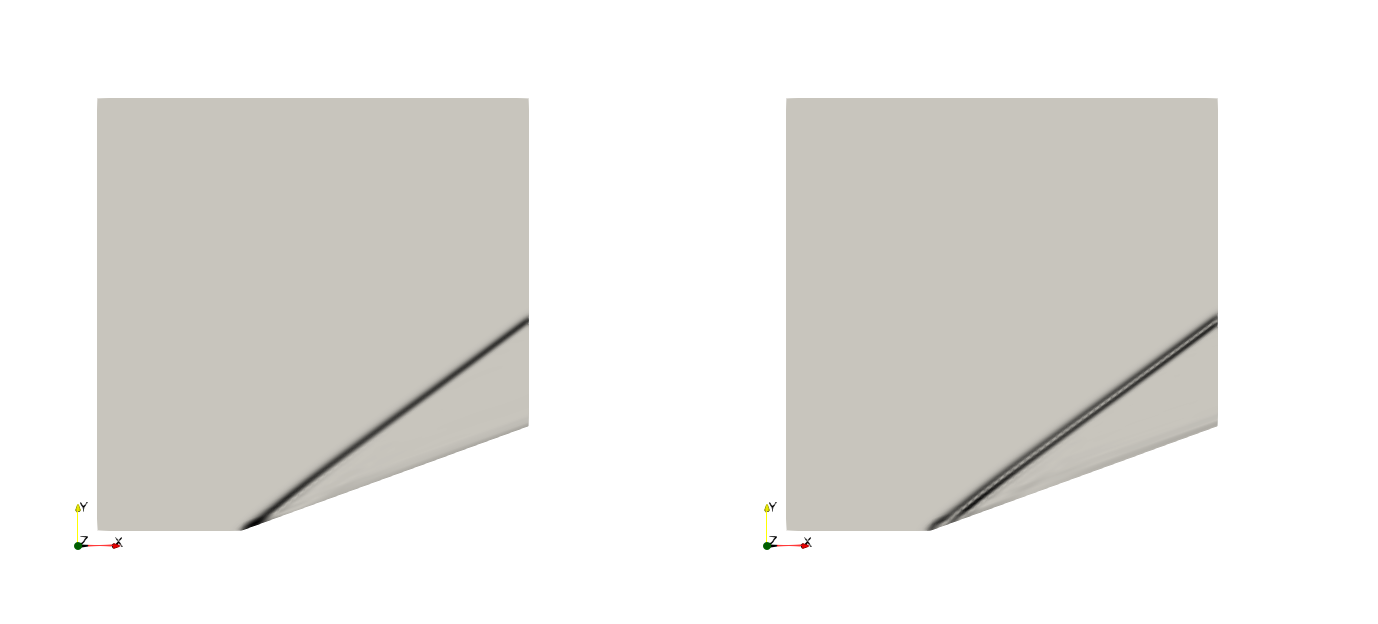Shock wave visualization. Left: numerical Schlieren. Right: numerical shadowgraph.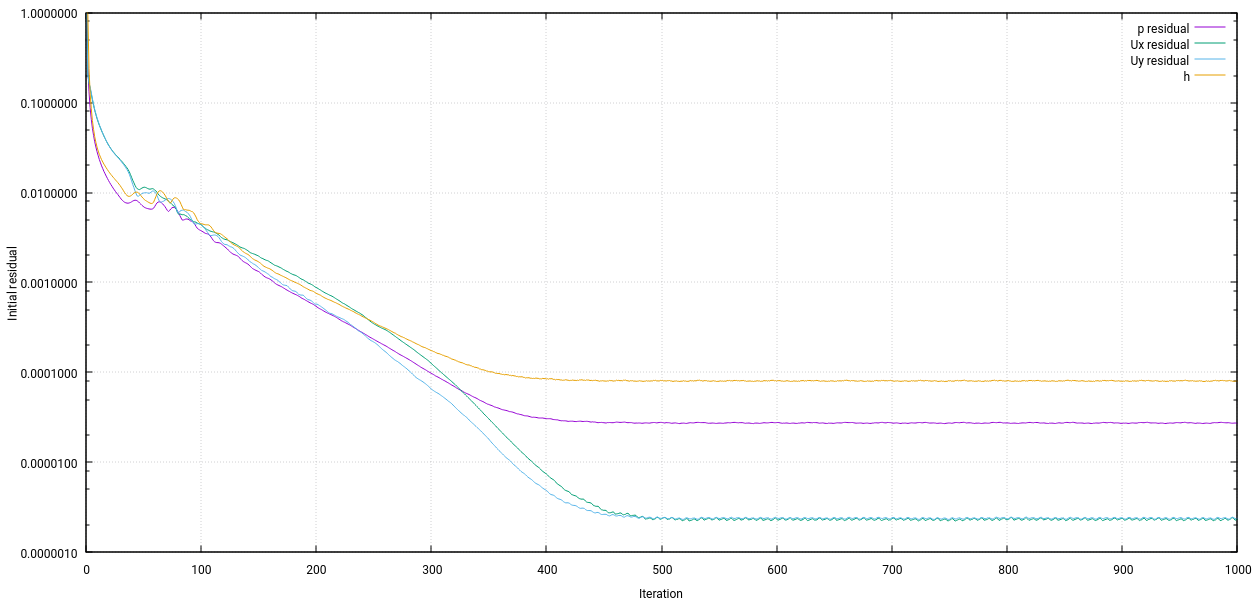Residuals behavior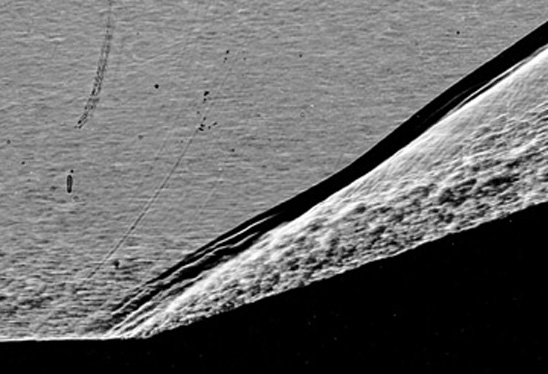The picture is a shadowgraph of Mach 3 airflow over an 18 degree compression corner which shows the shock wave/turbulent boundary-layer interaction (from G. Settles, PhD thesis, Princenton, 1975)

Wedge angle $$M_1$$$$\beta$$ exact$$\beta$$ OpenFOAMPercent Error (%)
$$20^{\circ}$$ 3 37.8 36.9 0.023

Wedge angle  $$M_1$$$$M_2$$ exact$$M_2$$ OpenFOAMPercent Error (%)
$$20^{\circ}$$ 3 1.988 1.999 0.005

warning  The exact values were extracted from plots illustrated in the references below.
We can not guarantee the accuracy or precision of this information.

References:

Modern compressible flow: with historical perspective. Third edition. McGraw-Hill.

Compressible aerodynamics calculator. http://www.dept.aoe.vt.edu/~devenpor/aoe3114/calc.html

Download here the case file.